# TN Board Class 12 Maths Important Questions And Solutions

Mathematics is a subject that requires problem-solving skills and also develops a love for the subject. Mastering in this subject will help students to better understand the subject like Physics and other sciences and don’t forget that Maths is used in our everyday life. Class 12 Maths important question will also help students to face various competitive exams like JEE, NEET and other engineering entrance exams. These important questions will help them to reveal areas on which they need to work on after solving each and every important question of Class 12 Maths. Students can solve these Tamil Nadu Board Class 12 Maths Important Questions for practice.

## TN Board Class 12 Mathematics Important Questions

Compared to any other subject, preparing for Mathematics is easier because it is totally based on numerical problems and a number of questions in Maths are repeated every year. Students can easily make an idea about the upcoming exam question by solving important questions of Class 12 Maths TN board. Class 12 TN Board Maths syllabus covers topics such as vector algebra, probability distributions, analytical geometry, etc. These important questions of Class 12 Maths are prepared by subject experts after analysing the previous year question paper or sample paper of Class 12 Maths.

There is a chance that these important questions of Class 12 Maths can be asked in 12th board exam that’s why we have focused to prepare you by providing different sets of important questions of Class 12 Maths. Students are advised to practice these important questions of Class 12 Maths after completion of their curriculum as they are ideally structured by giving importance on different topics.

### Download TN Board Class 12 Maths Important Questions And Solutions PDF

Question 1: Prove that the function f (x) = x2 – x + 1 is neither increasing nor decreasing in [- 1, 1].

Solution:

f (x) = x² – x + 1

f’(x) = 2x – 1

If f’(x) = 0, then, x = 1 / 2

The point x = 1 / 2 divides the interval (-1, 1) into two disjoint intervals (-1, 1 / 2) and [1 / 2, 1).

Consider the interval (-1, 1 / 2)

f ‘ (x) = 2x – 1 < 0

f is decreasing in the interval (-1,1 / 2)

Consider the interval [1 / 2, 1)

f ‘ (x) = 2x – 1 > 0

f is increasing in the interval [1 / 2, 1)

Hence, f is neither strictly increasing and decreasing in the interval (-1, 1).

Question 2: Prove that the cube roots of unity form an abelian group under multiplication.

Solution:

The cube roots of unity are (1, ω, ω²).

Consider G to be the set (1, ω, ω²).

(a) Closure: ∀ a, b ∈ G, a * b also ∈ G.

(b) Associative: ∀ a,b and c ∈ G,

a * (b * c) = (a * b) * c

(c) Identity: ‘e’ is called the identity element of the group if ∀ a ∈ G, a * e = e = e * a.

From the table, it is clear that e = 1 is the identity.

(d) Inverse: If ∀ a ∈ G, ∃ a, b such that a * b = e, then b is called the inverse of a.

The inverse of 1 is 1, the inverse of ω is ω² and the inverse of ω² is ω.

All the four properties of a group are satisfied. Hence, G is a group.

From the table, ∀ a, b ∈ G, a * b = b * a

So, G is commutative.

A commutative group is called an abelian group.

× 1 ω ω²

===============

1 1 ω ω²

ω ω ω² 1

ω² ω² 1 ω

The cube roots of unity form a finite abelian group under multiplication.

Question 3: Solve by matrix inversion method 2x − y = 7 and 3x − 2y = 11.

Solution: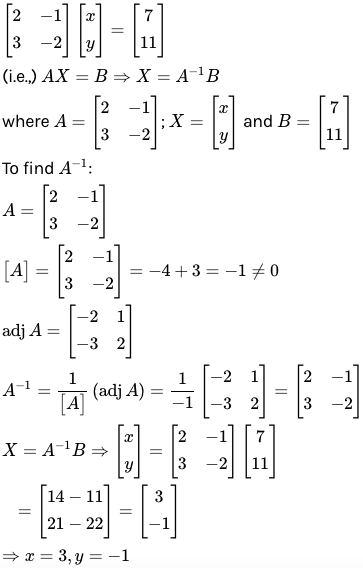Question 4: What is the radius of the circle in which the sphere x2 + y2 + z2 + 2x – 2y – 4z – 19 = 0 is cut by the plane x + 2y + 2z + 7 = 0?

Solution:

x2 + y2 + z2 + 2x – 2y – 4z – 19 = 0

Radius of the sphere = r = √12 + (-1)2 + (-2)2 + 19 = 5

Center of the sphere is C = (−1, 1, 2)

Distance of the given plane from the centre is CP = [−1 + 2 + 4 + 7] / √1 + 4 + 4

= 4

The radius of the circle is √r2 – CP2

= √52 – 42

= √25 – 16

= √9

= 3

Question 5: Find the vector and cartesian equations to the plane through the point (–1, 3, 2) and perpendicular to the plane x + 2y + 2z = 5 and 3x + y + 2z = 8.

Solution:

The normal vector to the given planes x + 2y + 2z = 5 and 3x + y + 2z = 8 are i + 2j + 2k and 3i + j + 2k. These vectors are parallel to the required plane.

The required plane passes through the point A (-1, 3, 2) and is parallel to the vectors u = i + 2j + 2k and v = 3i + j + 2k.

The required equation is r = a + su + tv

r = (-i + 3j + 2k) + s (i + 2j + 2k) + t (3i + j + 2k)

The cartesian form is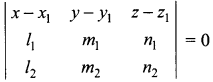.

The equation of the plane is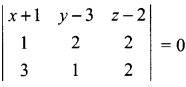(x + 1) (4 – 2) – (y – 3) (2 – 6) + (z – 2) (1 – 6) = 0

2x + 2 + 4y – 12 – 5z + 10 = 0

2x + 4y – 5z = 0

Question 6: The path of a ship can be described by a hyperbolic model centred at the origin, relative to two stations on the shore 168 miles apart that are located at the foci. If the ship is 40 miles south of the centre of the hyperbola, find the equation of the hyperbola.

Solution:

The focus of hyperbola is represented by two stations that are equidistant from the centre is 168 miles.

The coordinates of focus are C (84, 0) and C’ (-84, 0).

The general equation of the hyperbola is,

x2 / A2 + y2 / B2 = 1

The coordinates of one vertex are (A, 0) and the other is (0, B).

B is the point where the ship is located.

C² = A² + B²

84² = A² + 40²

A² = 7056 -1600

A² = 5456

A = 73.86

The equation of the path of the ship which is in the form of hyperbola is (x2 / 5456 + y2 / 1600) = 1.

Question 7: Derive the formula for the volume of a cylinder with radius ‘r’ and height ‘h’ by using integration.

Solution: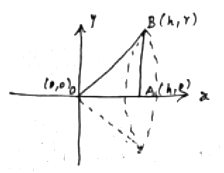Consider a triangle AOB with vertices O (0, 0), A (h, 0), B (h, r).

Equation of OB, y = (r / h) x

V = 𝛑 ∫0h y2 dx

= 𝛑 ∫0h (r2 / h2) x2

= 𝛑r2 / h2 [x3 / 3]0h

= 𝛑r2 / h2 [h3 / 3]

= (1 / 3) 𝛑r2h cubic units

Question 8: A bank pays interest by continuous compounding that is by treating the interest rate as the instantaneous rate of change of principal. Suppose in an account interest accrues at 8% per year compounded continuously. Calculate the percentage increase in such an account over one year.

Solution:

Let P0 be the initial amount and P be the amount at any time t.

dP / dt = 8P / 100

dP / dt = 2P / 25

dP / P = (2 / 25) dt

On integrating both sides,

log P = (2 / 25) t + c — (1)

P = P0 at t = 0

log P0 = 0 + c

c = log P0

Substitute the value of c in (1),

log P = (2 / 25) t + log P0

log (P / P0) = (2 / 25) t

e(2/25)t = P / P0

Amount after 1 year

e(2/25) = P / P0

e0.08 = P / P0

1.0833 = P / P0

P = 1.0833 P0

Percentage increase = [(P – P0) / P0] * 100

= 0.0833 * 100

= 8.33%

Question 9: If n is a positive integer, prove that [(1 + sinθ – i cosθ) / (1 + sinθ + i cosθ)]n = cos n (𝛑 / 2 – θ) – i sin n (𝛑 / 2 – θ).

Solution:

Let Z = sinθ – i cosθ

|Z| = 1

(1 / Z) = (bar Z)

(1 / Z) = sinθ + i cosθ

LHS = [(1 + sinθ – i cosθ) / (1 + sinθ + i cosθ)]n

= [(1 + Z) / (1 + (1 / Z))]n

= [((1 + Z) / (Z + 1) / Z)]n

= Zn

= (sinθ – i cosθ)n

= [cos (𝛑 / 2 – θ) – i sin (𝛑 / 2 – θ)]n

= cos n (𝛑 / 2 – θ) – i sin n (𝛑 / 2 – θ)

= RHS

Question 10: Find the length of the latus rectum of an ellipse 4x2 + 9y2 – 24x + 36y – 72 = 0.

Solution:

4x2 + 9y2 – 24x + 36y – 72 = 0

⇒ ( 4x2 – 24x ) + ( 9y2 + 36y ) – 72 = 0

⇒ 4( x2 – 6x ) + 9( y2 + 4y ) – 72 = 0

⇒ 4[ x2 – 6x + 9 ] + 9[ y2 + 4y + 4 ] = 144

⇒ 4( x – 3 )2 + 9( y + 2 )2 = 144

⇒ {( x – 3 )2 / 36} + {( y + 2 )2 / 16 } = 1

⇒ {( x – 3 )2 / 62} + {( y + 2 )2 / 42 } = 1

⇒ a = 3 and b = 2

Therefore, the length of the latus rectum of an ellipse is given as:

= 2b2 / a

= 2(2)2 / 3

= 2(4) / 3

= 8 / 3

Hence, the length of the latus rectum of ellipse is 8 / 3.

Question 11: Out of 13 applications for a job, there are 8 men and 5 women. It is decided to select 2 persons for the job. Find the probability that at least one of the selected people will be a woman.

Solution:

The probability that none of the women will be selected = the probability of both men selected.

The probability of both men selected = 8C2 / 13C2

= (8 * 7) / (13 * 12)

= 14 / 39

The probability of at least one woman will be selected = 1 – (14 / 39)

= 25 / 39

Question 12: Altitudes of a triangle are concurrent prove by vector method.

Solution: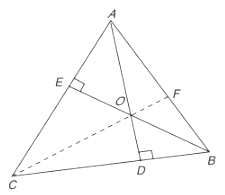Let the altitudes AD and BE intersect at O.

Join CO and produce to meet AB in F.

Let OA = a, OB = b, OC = c.

The vector a is perpendicular to BC = OC – OB = c – b

a (c – b) = 0

a . c – a . b = 0

a . c = a . b —– (1)

The direction OB is perpendicular to AC.

b . (c – a) = 0

b . c = a . b —- (2)

From (1) and (2),

a . c = a . b = b . c

c . a – c . b = 0

c . (a – b) = 0 —- (3)

(a – b) = OA – OB = BA

From equation 3, vector c is perpendicular to vector BA; but vector c is a vector in the direction of vector OC.

Hence, vector OC is perpendicular to vector AB, that is, CF is the third altitude of the triangle through C.

Hence, the three altitudes are concurrent at O.

Question 13: Find the axes, centre, focus and directrix of the parabola y2 + 6y + 8x + 25 = 0.

Solution:

y2 + 6y + 8x + 25 = 0

y2 + 6y = – 8x – 25

y2 + 6y + 9 = – 8x – 25 + 9

(y + 3)2 = – 8x – 16

(y + 3)2 = – 8 (x + 2)

On comparing this equation,

(y – b)2 = 2p (x – a)

2p = – 8

p = – 4

The vertex is (a, b) = (- 2, -3)

The focus is (a + p / 2, b) = (- 4, -3)

The directrix is x = a – p / 2

x = – 2 + 2 = 0

Question 14: The orbit of the earth around the sun is elliptical in shape with the sun at a focus. The semi-major axis is of length 92.9 million miles and eccentricity is 0.017. Find how close the earth gets to the sun and the greatest possible distance between the earth and the sun.

Solution: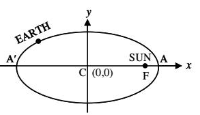The semi-major axis is CA = a = 92.9 million miles

e = 0.017

The closest distance of the earth from the sun = FA and the farthest distance of the earth from the sun = FA’.

CF = ae = 92.9 * 0.017

FA = CA – CF

= 92.9 – 92.9 * 0.017

= 92.9 [1 – 0.017]

= 91.3207 million miles

FA’ = CA’ + CF

= 92.9 + 92.9 * 0.017

= 92.9 [1 + 0.017]

= 94.4793 million miles

Question 15: Prove that the perpendicular bisectors of sides of a triangle are concurrent by vector method.

Solution: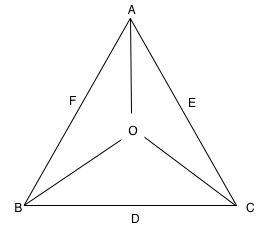Let ABC be a triangle.

D, E F are midpoints of BC, AC and AB.

Let O (origin) be the point of intersection of the perpendicular bisectors OD and OE.

Let OA = a, OB = b and OC = c.

Since D is the midpoint of BC, from section formula,

OD = OB + OC / 2 = b + c / 2

E is mid point of AC,

⇒ OE = a + c / 2 and OF = a + b / 2

But OD is perpendicular bisector to BC,

⇒ OD . BC = 0

⇒ b + c / 2 . (c − b) = 0

⇒ |c|2 − |b|2 = 0 ………… (i)

Similarly OE is ⊥ bisector to AC.

⇒ OE . AC = 0

⇒ c + a2 . (c − a) = 0

⇒ |c|2 − |a|2 = 0……………. (ii)

Subtracting (i) – (ii),

⇒ (|c|2 − |b|2) − (|c|2 − |a|2) = 0

⇒ |a|2 − |b|2 = 0 ……….. (iii)

⇒ OF . AB = a + b / 2 . (b − a)

= |b|2 − |a|2 / 2 = 0 from (iii)

⇒ OF . AB = 0

⇒ OF is perpendicular to AB.

⇒ OF is also a perpendicular bisector.

All three perpendicular bisectors are concurrent.

Question 16: A Kho-Kho player in a practice session while running realises that the sum of the distances from the two Kho-Kho poles from him is always 8m. Find the equation of the path traced by him if the distance between the poles is 6m.

Solution: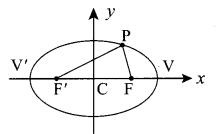FP + F’P = 4

2a = 8

a = 4

FF’ = 2ae = 6

ae = 3

e = ae / a = 3 / 4

b2 = a2 (1 – e2) = 16 [1 – (9 / 16)] = 7

The equation of the path is an ellipse whose equation is (x2 / 16) + (y2 / 7) = 1.

Question 17: The life of army shoes is normally distributed with mean 8 months and a standard deviation of 2 months. If 5000 pairs are issued, how many pairs would be expected to need replacement after 12 months?

Solution:

Mean = μ = 8

Standard deviation = σ = 2

Number of pair of shoes = 5000

Total months = x = 12

z = x – μ / (σ)

= (12 – 8) / 2

= 2

Area (z ≥ 2) = 0.0228

The number of pairs whose life is more than 12 months (z > 2)

= 5000 * 0.0228

= 114

Replacement after 12 months = 5000 – 114 = 4886 pairs of shoes

Question 18: Prove that the solution of the differential equation : (5D2 − 8D − 4)y = 5e(-⅖x) + 2ex + 3 is y = Ae2x + Be(-⅖x) – (5 / 12) xe(-⅖x) – (2 / 7) ex – (3 / 4).

Solution:

C.F = A𝑒2𝑥 + B𝑒 (−2/5)𝑥

P.I1 = (- 5 / 12) x𝑒 (−2/5)𝑥

P.I2 = (- 2 / 7) 𝑒𝑥

P.I3 = – 3 / 4

General solution y = C.F + P.I1 + P.I2 + P.I3

= A𝑒2𝑥 + B𝑒 (−2/5)𝑥 + (- 5 / 12) x𝑒 (−2/5)𝑥 + (- 2 / 7) 𝑒𝑥 (- 3 / 4)